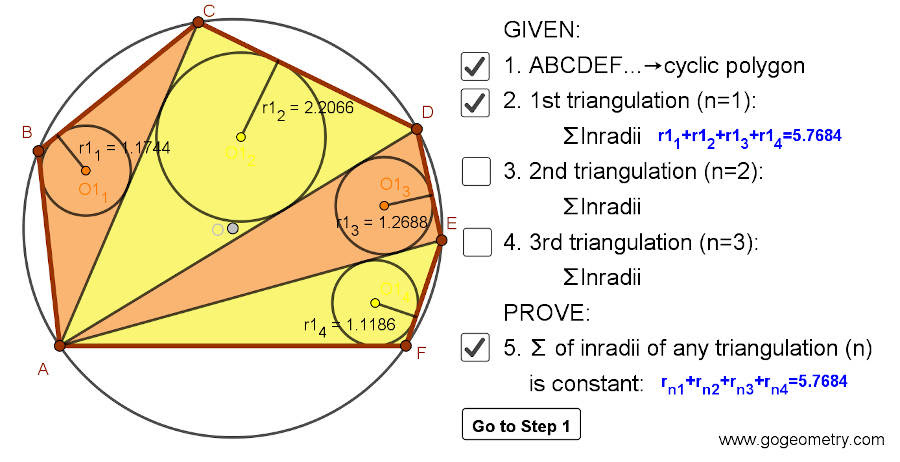## Friday, May 15, 2020

### Dynamic Geometry 1480: Japanese Theorem for Cyclic Polygon, Triangulation, Non-intersecting Diagonals, Sum of Inradii, Invariant, Step-by-step Illustration

Interactive step-by-step animation using GeoGebra. Post your solution in the comment box below.
Level: Mathematics Education, High School, Honors Geometry, College.

Details: Click on the figure below.#### 1 comment:

1.http://gogeometry.com/school-college/5/p1452-cyclic-quadrilateral-sangaku-japanese-theorem-geogebra-ipad.htm

Denote [XYZ]= inradius of triangle XYZ
Per the result of problem 1452. For any cyclic quadrilateral ABCD we always have
[ABD]+[BDC]=[ADC]+[ACB]
For 2nd triangulation of problem 1480:
In quadrilateral BAFE, apply the result of problem 1452 we have
[AFB]+[BFE]= [AFE]+[ADE]+[ABD]-[BDE]…. (1)
Similarly, for quadrilateral BEDC we have
[BED]+[BDC]=[AED]+[ADC]+[ACB]- [AEB]….(2)
Add (1) to (2) and note that [ABD]+[AED]=[BDE]+[AEB]
We get [AFB]+[BFE]+[BED]+[BDC]=[AFE]+[AED]+[ADC]+[ACB]
So summation of all inradii of 2nd triangulation and 1st triangulation are equal=
With similar way we will have the summation of all inradii of 3rd triangulation and 1st triangulation are also equal